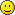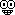# Count 1 - 1000

Go to page :1, 2, 3#### orobouRena Veteranyay 26 merry after xmas

#### ChoculaTWENNY SE7EN

_________________#### orobouRena Veteran28

#### Chocula29!_________________#### orobouRena Veteransweet 30Helper[(3x10)+1)] = THIRTY-ONE!!!!!!!!!!!!!!!#### orobouRena Veteraniam dirty too =D xDHelperThen I'm a dirty tree#### orobouRena Veterandirty forHelper[(25/5)*(7)] = Thirty-Five!!! 35

#### Chocula[(5*3)/5]+[(6*2)*2]+[(3*2)/6]+[2(2+2)] = 36. (:

_________________#### Njord333/3^2 :)

so many threes! I'm dirty seven. :)Helper(76/x)=2 The value of x is 38!!!!

#### Chocula5x + 7x + 5 - 10x + 2x^2 * 2 + (squareroot of 25)
= 39; When x is 2.

P.S. I DON'T FOLLOW PEMDAS, K? K.

Last edited by Chocula on Wed Apr 21, 2010 2:36 am; edited 1 time in total

_________________HelperChocula wrote:5x + 7x + 5 - 10x + 2x^2 * 2 + (squareroot of 25)
= 39; When x is 2.

= 5(2) + 7(2) + 5 - 10(2) + 2(2)^2 * 2 + (square root of 25)
= 10 + 14 + 5 - 20 + 2(4) * 2 + 5
= 29 - 20 + 16 + 5
= 29 - 41
= -12 in accordance to PEMDAS ( Parenthesis, Exponent, Multiplication, Division, Addition and Subtraction)

Anyway~ LIFE STARTS AT 40!!!!!!

#### Njord10(4).

#### ChoculaChocula wrote:5x + 7x + 5 - 10x + 2x^2 * 2 + (squareroot of 25)
= 39; When x is 2.

= 5(2) + 7(2) + 5 - 10(2) + 2(2)^2 * 2 + (square root of 25)
= 10 + 14 + 5 - 20 + 2(4) * 2 + 5
= 29 - 20 + 16 + 5
= 29 - 41
= -12 in accordance to PEMDAS ( Parenthesis, Exponent, Multiplication, Division, Addition and Subtraction)

Anyway~ LIFE STARTS AT 40!!!!!!

Oh, Okay. I didn't use PEMDAS. Kinda lazy.

Anyway, 42.

_________________HelperMy AGE! 43!

Just kidding~#### orobouRena Veteran44

#### Njord(3^2)(5)

#### orobouRena Veteran46

#### Chocula47.

_________________#### orobouRena Veteran48

#### ChoculaFOUR T NINE.

_________________#### orobouRena Veteranfiffti

Go to page :1, 2, 3